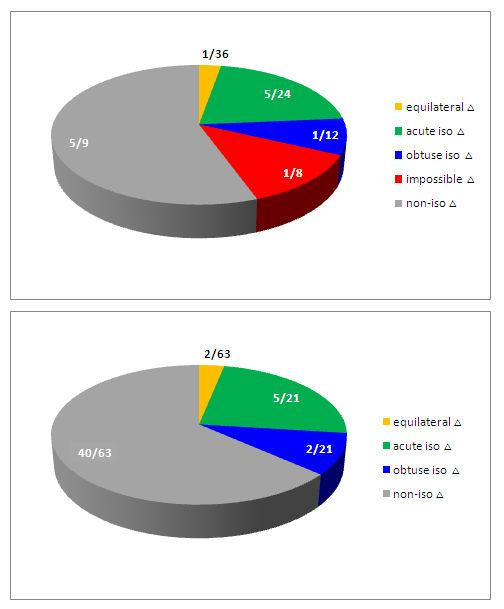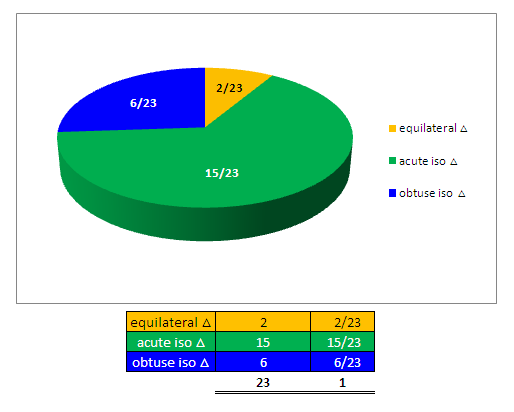BrainDen.com - Brain Teasers
• 0

Question

If the side of a triangle is dicided by throwing a dice 3 times. Then finding probability of forming Isoceles Triangle, is

Recommended Posts

• 0

3 in 20

Share on other sites
• 0

This sounds like it might be a homework problem, so I won’t flat out answer it but will give a hint on how to approach it.

Spoiler

It becomes a lot easier if you solve for the probability that you roll the dice three times and NOT get an isosceles triangle. In other words: what's the probability that you roll three times, and on each throw you roll a number that hasn't appeared yet?

Share on other sites
• 0
Posted (edited)

In a nutshell ...

Spoiler

There are 216 possible outcomes with three dice forming ...

Equilateral triangles: 6/216 (1/36)

No isosceles triangles: 120/216 (5/9)

Acute isosceles triangles: 45/216 (5/24)

Obtuse isosceles triangles: 45/216 (5/24)

All isosceles triangles (including equilateral triangles): 96/216 (4/9)

Edited by rocdocmac
typo

Share on other sites
• 0
Posted (edited)

A couple of questions:

Is a side of the triangle determined by a single die roll?  Or do you sum up three rolls per side?

Are non-possible triangles considered valid solutions?  e.g. A roll of 1,1,6 might appear isosceles, but would not be a valid triangle.

Edited by BobbyGo

Share on other sites
• 0
Posted (edited)
On 5/8/2019 at 10:57 PM, Thalia said:

Excluding equilateral triangles

Hide contents

2/9

7/24 without equilateral

3/8 with equilateral

Both excluding impossible triangles.

Edited by Thalia

Share on other sites
• 0

This gets tricky with rolls like (1, 1, 6) that look isosceles but don't actually form triangles.

How would you handle such rolls? Would you omit rolls that don't form a triangle from analysis (equivalent to saying that if you get a roll that doesn't form a valid triangle then roll again), or count them as a failure to form an isosceles triangle? And would you consider a "straight line" throw like (3, 3, 6) to be a valid triangle albeit with zero area or nah?

Share on other sites
• 0
Posted (edited)

Revised ...

Spoiler

I was way out with my first attempt!

Equilateral (EL) = 2/72 = (1/36)

Acute isosceles (AI) =  16/72 (2/9)

Obtuse isosceles (OI) =  5/72

Impossible triangles = 9/72 (1/8)

Normal triangles = 40/72 (5/9)

Total isosceles (AI+OI) = 18/72 (1/4)

Including equilateral (AI+OI+EL) = 23/72

Edited by rocdocmac
typo

Share on other sites
• 0
Posted (edited)

Please ignore previous submission ...

Spoiler

Here's a visual representation including and excluding "impossible" triangles

You can make your own subtotals!Edited by rocdocmac

Share on other sites
• 0
On 5/11/2019 at 4:26 PM, Thalia said:

Hide contents

7/24 without equilateral

3/8 with equilateral

Both excluding impossible triangles.

Well, I seem to have done some weird math when adding equilateral. New result including equilateral:

23/72

I think rocdocmac and I agree on the figure including equilateral now but we seem to have diverged on the other figure...

Share on other sites
• 0

My revised answers sent in previously were not all correct. These should be fine ...

Spoiler

With impossible triangles/straight-line cases:

Equilateral (EL) = 2/72 (1/36)

Acute isosceles (AI) =  15/72 (5/24)

Obtuse isosceles (OI) =  6/72 (1/12)

Impossible triangles (including "straight line" cases) = 9/72 (1/8)

Normal non-isosceles triangles = 40/72 (5/9)

Total isosceles (AI+OI) = 21/72 (7/24)

Including equilateral (AI+OI+EL) = 23/72﻿

Without impossible triangles/straight-line cases:

Equilateral (EL) = 2/63

Acute isosceles (AI) =  15/63 (5/21)

Obtuse isosceles (OI) =  6/63 (2/21)

Normal non-isosceles triangles = 40/63

Total isosceles (AI+OI) = 21/63 (1/3)

Including equilateral (AI+OI+EL) = 23/63﻿

Share on other sites
• 0
Posted (edited)

Excluding straight lines, impossible triangles and non-isosceles triangles, the distribution of all isosceles triangles is as follows ...

SpoilerEdited by rocdocmac
typo

Join the conversation

You can post now and register later. If you have an account, sign in now to post with your account.×   Pasted as rich text.   Paste as plain text instead

Only 75 emoji are allowed.

×   Your previous content has been restored.   Clear editor

×   You cannot paste images directly. Upload or insert images from URL.

×